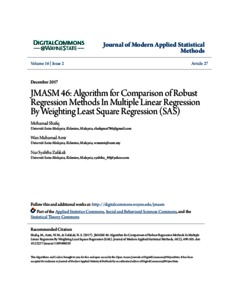# JMASM 46: Algorithm for comparison of robust regression methods in multiple linear regression by weighting least Square Regression

Mohd Ibrahim, Mohamad Shafiq and Wan Ahmad, Wan Muhamad Amir and Zafakali, Nur Syabiha (2017) JMASM 46: Algorithm for comparison of robust regression methods in multiple linear regression by weighting least Square Regression. Journal of Modern Applied Statistical Methods, 16 (2). pp. 490-505. ISSN 1538−9472Preview
PDF

## Abstract

The aim of this study is to compare different robust regression methods in three main models of multiple linear regression and weighting multiple linear regression. An algorithm for weighting multiple linear regression by standard deviation and variance for combining different robust method is given in SAS along with an application.

Item Type: Article (Journal) 8915/72210 Multiple linear regression, robust regression, M, LTS, S, MM estimation Q Science > QA Mathematics Kulliyyah of DentistryKulliyyah of Dentistry > Department of Paediatric Dentistry and Dental Public Health Dr Mohamad Shafiq Mohd Ibrahim 16 May 2019 14:24 16 May 2019 14:24 http://irep.iium.edu.my/id/eprint/72210View Item